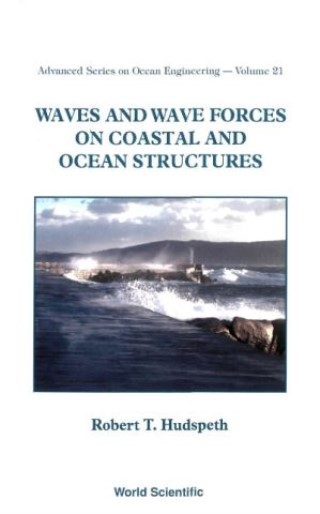## Waves And Wave Forces On Coastal And Ocean Structures• Description
• Author
• Info
• Reviews

### Description

This book focuses on: (1) the physics of the fundamental dynamics of fluids and of semi-immersed Lagrangian solid bodies that are responding to wave-induced loads; (2) the scaling of dimensional equations and boundary value problems in order to determine a small dimensionless parameter ε that may be applied to linearize the equations and the boundary value problems so as to obtain a linear system; (3) the replacement of differential and integral calculus with algebraic equations that require only algebraic substitutions instead of differentiations and integrations; and (4) the importance of comparing numerical and analytical computations with data from laboratories and/or nature.